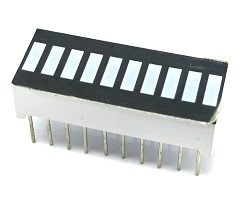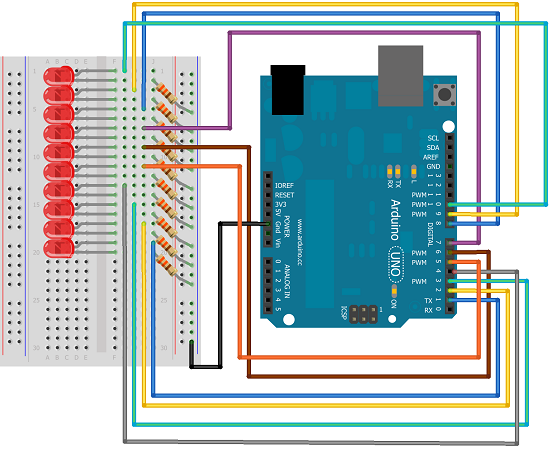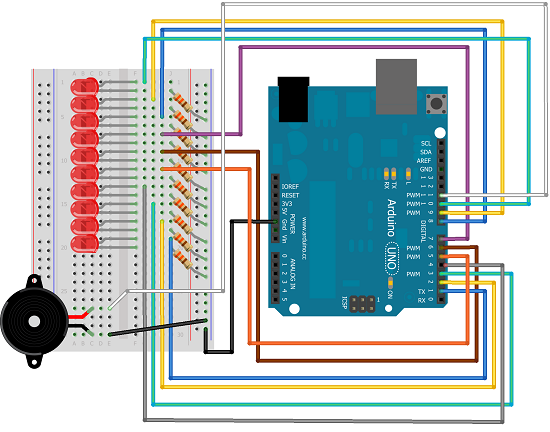# Bar Graph

## Introduction

This project explores a couple of ways of simulating and using a bar graph with the Arduino. Bar graph components like the following one can be used,This type of bar graph is basically just 10 LEDs in a row with anodes on one side of the component and cathodes on the other.

The same thing can be created by just placing 10 LEDs on a breadboard. If you have LEDs smaller than 5mm, you may be able to get them closer together on the breadboard. If not, just space out as you see in the diagrams.

## You Will Need

• 10 x LEDs or 1 x 10 Segement Bar Graph
• 10 x 330 Ohm Resistors
• 10K Trimmer Potentiometer
• 1 x Piezo Buzzer

## Making The Circuit - Bar Graph

The first job is to connect up all of those LEDs and resistors to make the bar graph.## Programming The Arduino - Bar Graph

Here is some code to test that the bar graph has been wired correctly.

```int ledPin[] = {1,2,3,4,5,6,7,8,9,10}; void setup() {   for (int i =0;i<10;i++)   {     pinMode(ledPin[i], OUTPUT);   } } void loop() {   upanddown();   delay(1000);   oneatatime();   delay(1000); } void alloff() {   for (int i =0;i<10;i++)   {     digitalWrite(ledPin[i], LOW);   }  } void allon() {   for (int i =0;i<10;i++)   {     digitalWrite(ledPin[i], HIGH);   }  } void showUpTo(byte numtoshow) {   alloff();   for (byte i = 0;i<numtoshow;i++)   {     digitalWrite(ledPin[i],HIGH);   }  } void upanddown() {   for (int i =0;i<10;i++)   {     digitalWrite(ledPin[i], HIGH);     delay(100);   }   for (int i=9;i>=0;i--)   {     digitalWrite(ledPin[i], LOW);     delay(100);   } } void oneatatime() {   for (int i =0;i<10;i++)   {     digitalWrite(ledPin[i], HIGH);     delay(100);     digitalWrite(ledPin[i], LOW);     delay(100);   }   } ```

## Making The Circuit - Trimpot

Adding a trimpot to control the bar graph allows the bar graph to be used as an indicator for the position of the potentiometer.## Programming The Arduino - Trimpot

```int ledPin[] = {1,2,3,4,5,6,7,8,9,10}; int potPin = A0; void setup() {   for (int i =0;i<10;i++)   {     pinMode(ledPin[i], OUTPUT);   } } void loop() { fromPot(); } void alloff() {   for (int i =0;i<10;i++)   {     digitalWrite(ledPin[i], LOW);   }  } void allon() {   for (int i =0;i<10;i++)   {     digitalWrite(ledPin[i], HIGH);   }  } void showUpTo(byte numtoshow) {   alloff();   for (byte i = 0;i<numtoshow;i++)   {     digitalWrite(ledPin[i],HIGH);   }  } void fromPot() {   int potvalue = analogRead(potPin);   byte show = map(potvalue,0, 1023, 0,10);   showUpTo(show); } void upanddown() {   for (int i =0;i<10;i++)   {     digitalWrite(ledPin[i], HIGH);     delay(100);   }   for (int i=9;i>=0;i--)   {     digitalWrite(ledPin[i], LOW);     delay(100);   } } void oneatatime() {   for (int i =0;i<10;i++)   {     digitalWrite(ledPin[i], HIGH);     delay(100);     digitalWrite(ledPin[i], LOW);     delay(100);   }   }```

## Making The Circuit - Buzzer

Now to make the buzzer and lights work together.## Programming The Arduino - Buzzer

The program includes definitions of the 10 natural notes that start with middle C. Each light represents a note and is switched on when the note is playing.

```int buzzerPin = 11; int tempo = 150; int ledPin[] = {1,2,3,4,5,6,7,8,9,10}; int notes[] = {262,294,330,349,392,440,494,523,587,659}; int tunelength = 14; int tune[] = {0,0,4,4,5,5,4,3,3,2,2,1,1,0}; int beats[] = {1, 1, 1, 1, 1, 1, 2, 1, 1, 1, 1, 1, 1, 2}; void setup() {   for (int i =0;i<10;i++)   {     pinMode(ledPin[i], OUTPUT);   }   pinMode(buzzerPin, OUTPUT); } void playTune() {   for (int i =0;i<tunelength;i++)   {     tone(buzzerPin, notes[tune[i]], beats[i]*tempo);     digitalWrite(ledPin[tune[i]], HIGH);     delay(beats[i]*tempo);     digitalWrite(ledPin[tune[i]], LOW);     delay(tempo/10);   } } void loop() {   playTune();   while(true){   }; } void alloff() {   for (int i =0;i<10;i++)   {     digitalWrite(ledPin[i], LOW);   }  } void allon() {   for (int i =0;i<10;i++)   {     digitalWrite(ledPin[i], HIGH);   }  } void showUpTo(byte numtoshow) {   alloff();   for (byte i = 0;i<numtoshow;i++)   {     digitalWrite(ledPin[i],HIGH);   }  }```

## Challenges

You could start by changing the tune in the buzzer version.

There are lots of other sensor values that you can use a bar graph with. A thermometer would be a starting point.

If you make 4 of the LEDs a different colour from the others, you have enough LEDs to do the output for a binary clock. (You'd be using 12hr format for the hour part of the time.) If you have access to a Real Time Clock module, you could use that to source your time components.Alternatively, you can look for the Time library on the Arduino web site and do a similar job without.